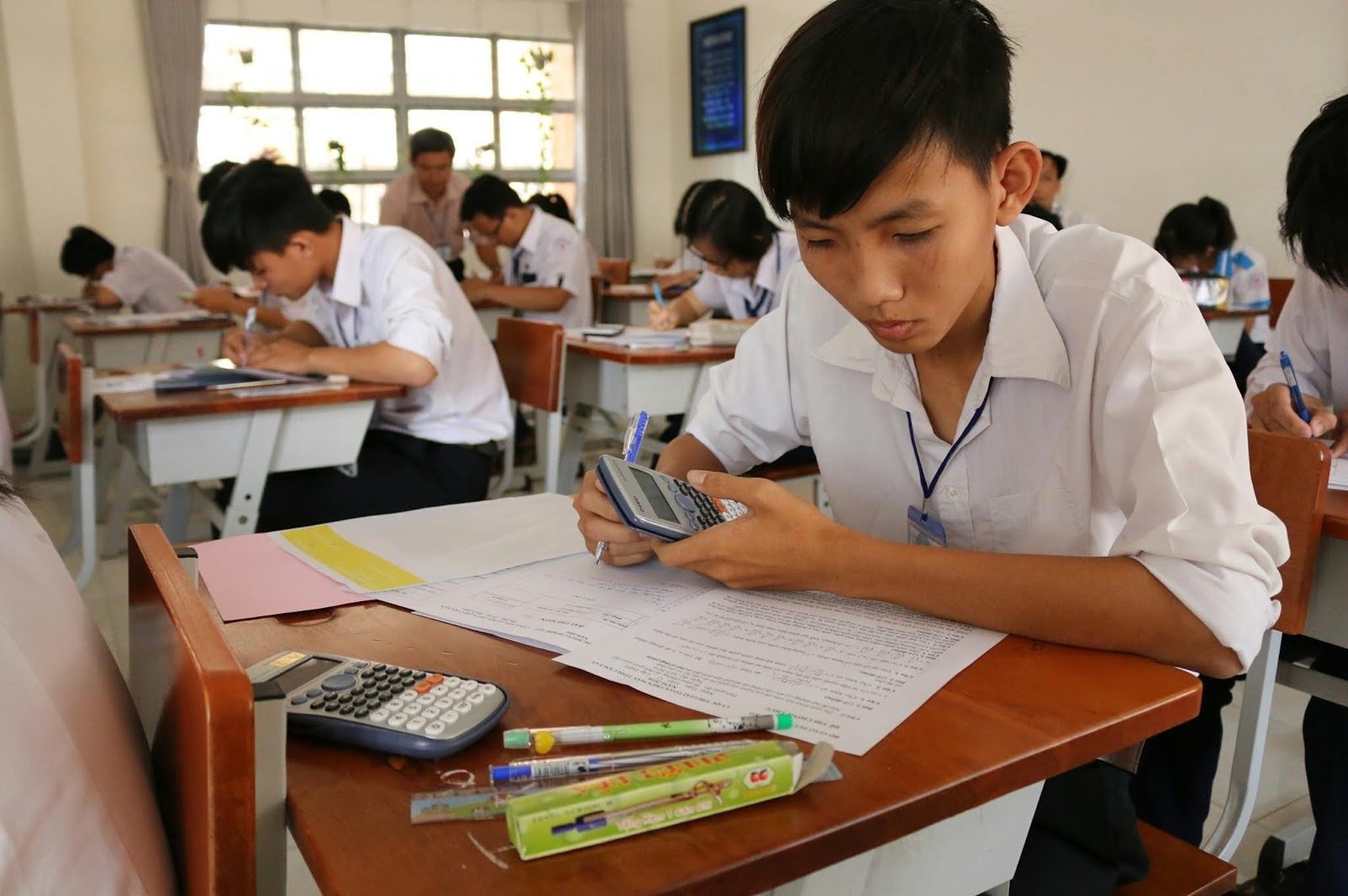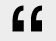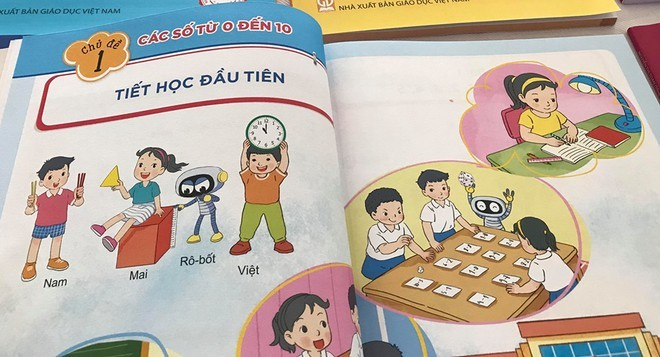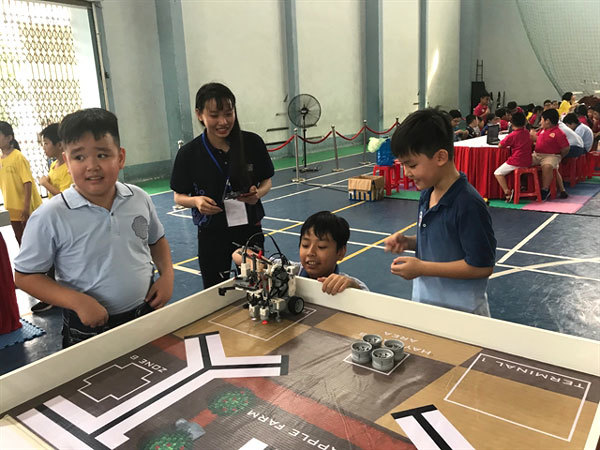Dr Ngo Hoang Long from the Hanoi University of Education recently gave a presentation themed ‘Probability and statistics in the new general education program’ at the Open Math Day some days ago.Long said probability and statistics is one of three important parts of the mathematics curriculum in the new general education program. Under the program, second graders will begin learning probability and statistics.

The information has worried parents. They look back on what they learned in the last year at high school and at university and believe that the subject would be beyond the capability of second graders.

In fact, probability and statistics have been familiar to general school students for many years. Under the current general education program, students study probability and statistics.

In the lesson about ‘dates and months’, second graders get familiar with factors and statistics. They have to solve some questions: how many Sundays are there in December and which are the days?In fact, probability and statistics have been familiar to general school students for many years. Under the current general education program, students study probability and statistics.

Meanwhile, third graders are required to measure length and enter the figures into tablets. Students also learn about probability and statistics when observing traffic to find out which hours traffic congestion occurs the most.

According to Do Duc Thai, chief editor of the new math program, many parents find the name of the subject - probability and statistics - too abstract, and therefore, fear the subject would be beyond students’ capability. However, he affirmed that there was no need to worry about this, because the knowledge will be provided in a simple and reasonable way.

Instead of requesting students to learn the definitions and concepts, students will be able to learn math through games and experience activities.

Education experts see probability and statistics as an important part of knowledge which can help promote the applicability of math.

“One not only needs to find information, but also to analyse and process information. The knowledge about probability and statistics will help students in particular and citizens in general make reasonable decisions,” Long said.

“Probability and statistics promote logical thinking, which helps people make decisions while considering the probabilities and possible consequences for each option. Learning probability and statistics, students will learn how to make decisions that can minimize risks,” he explained.

Dr Chu Cam Tho from the Vietnam Education Science Institute commented that one of the strongest points of the new math program is the improvement of the teaching of applied math, including probability and statistics.

Chi Mai#### Vietnam to teach probability and statistics to second graders

Under the new general education program, the mathematics curriculum will focus on three major parts: arithmetic, algebra and analytical factors; geometry and measurement; and statistics and probability.#### Students at HCM City primary schools win top prize at Robothon

Students at Le Ngoc Han and Luong The Vinh primary schools won the top prizes in the annual Robothon Contest 2019 for students at primary schools throughout HCM City held on October 20.